# Classical physics and Quantum theory What was classical

• Slides: 15Classical physics and Quantum theoryWhat was classical physics? • Classical physics is physics up to the end of the nineteenth century. • It includes Newtonian physics and Maxwells equations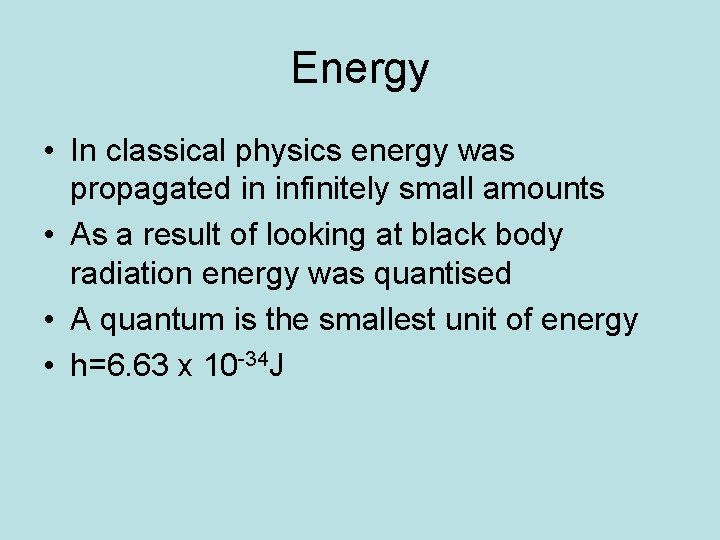Energy • In classical physics energy was propagated in infinitely small amounts • As a result of looking at black body radiation energy was quantised • A quantum is the smallest unit of energy • h=6. 63 x 10 -34 J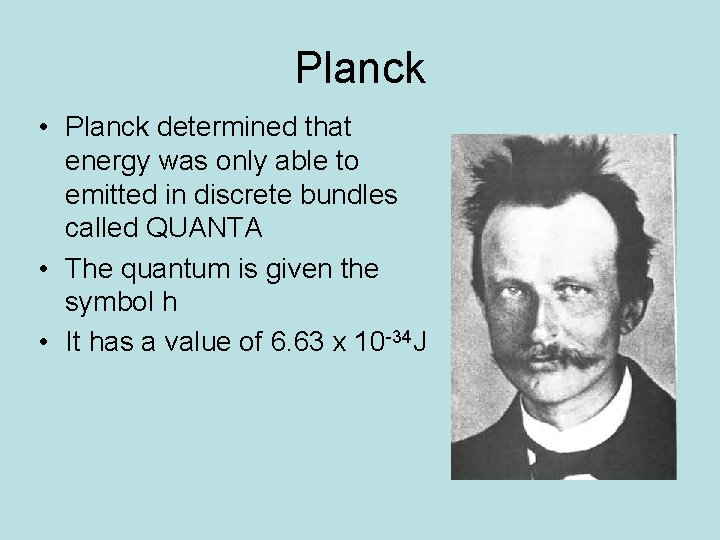Planck • Planck determined that energy was only able to emitted in discrete bundles called QUANTA • The quantum is given the symbol h • It has a value of 6. 63 x 10 -34 JQuantum energy is like the potential energy gained going up steps. Each step has a particular height and energy and there is no halfway point between each energy level.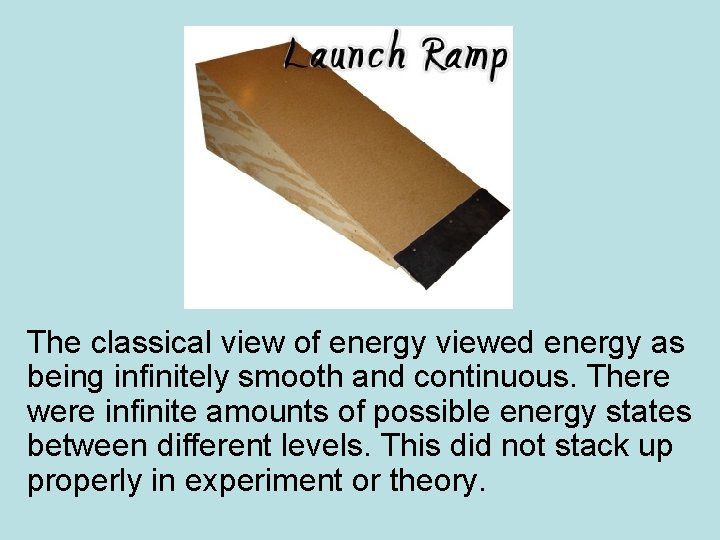The classical view of energy viewed energy as being infinitely smooth and continuous. There were infinite amounts of possible energy states between different levels. This did not stack up properly in experiment or theory.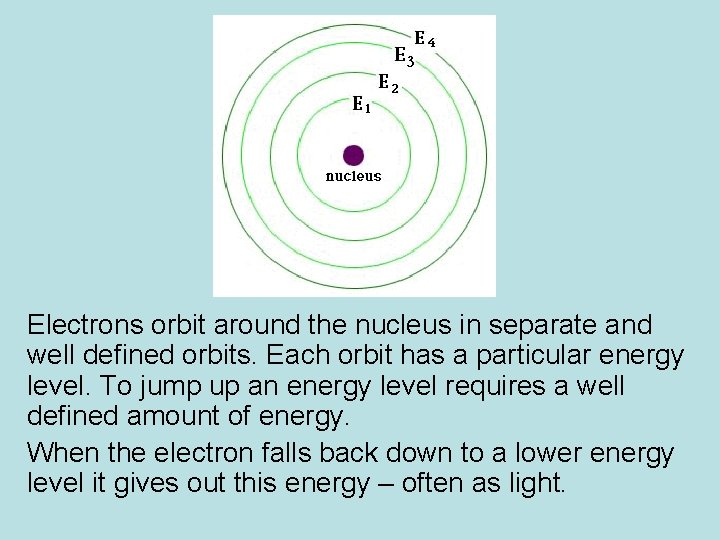Electrons orbit around the nucleus in separate and well defined orbits. Each orbit has a particular energy level. To jump up an energy level requires a well defined amount of energy. When the electron falls back down to a lower energy level it gives out this energy – often as light.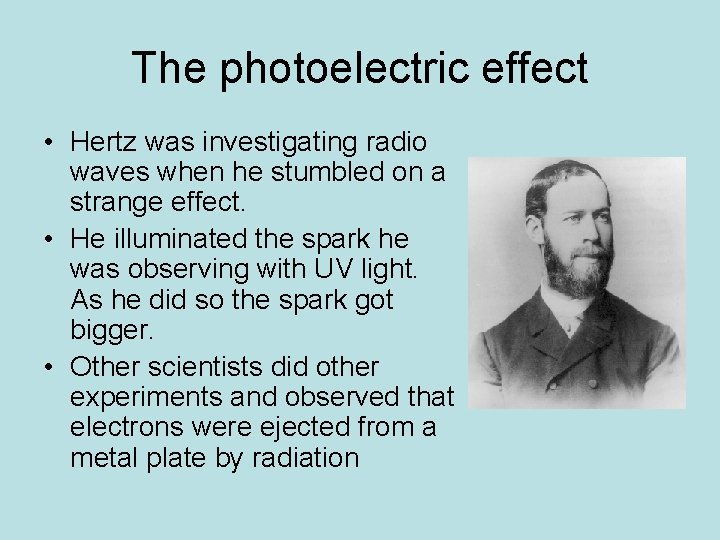The photoelectric effect • Hertz was investigating radio waves when he stumbled on a strange effect. • He illuminated the spark he was observing with UV light. As he did so the spark got bigger. • Other scientists did other experiments and observed that electrons were ejected from a metal plate by radiation• Philip von Lenard observed that individual electrons could be repelled with electric charge • There was a minimum charge needed to repel the electrons – under that minium threshold the electrons were not repelled • Lenard observed • Doubling the light doubled the number of eletcrons • There was no change of energy as the light intensity increased • The maximum energy of electrons depended on the frequency of lightClassical physics was stumped!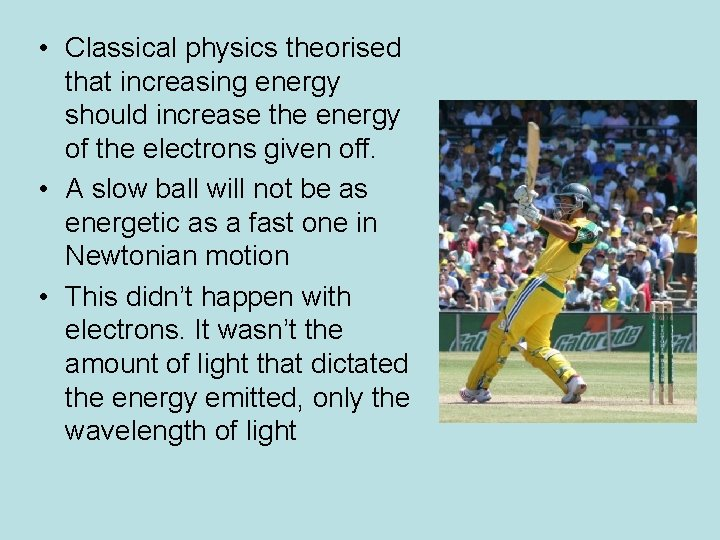• Classical physics theorised that increasing energy should increase the energy of the electrons given off. • A slow ball will not be as energetic as a fast one in Newtonian motion • This didn’t happen with electrons. It wasn’t the amount of light that dictated the energy emitted, only the wavelength of light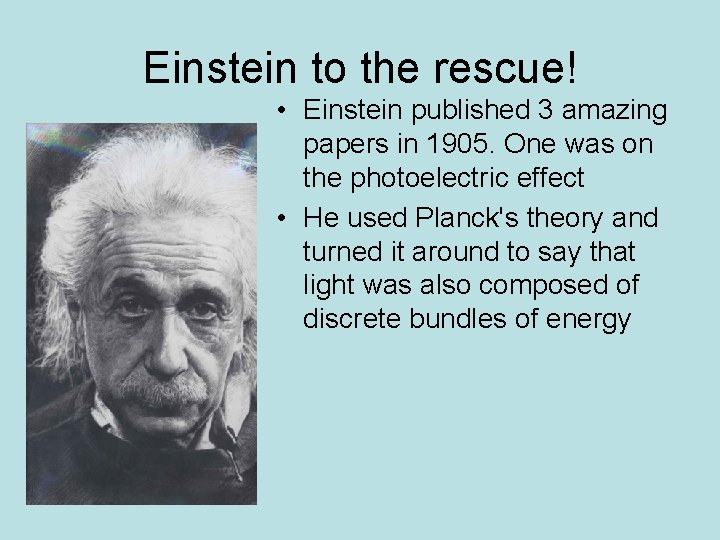Einstein to the rescue! • Einstein published 3 amazing papers in 1905. One was on the photoelectric effect • He used Planck's theory and turned it around to say that light was also composed of discrete bundles of energy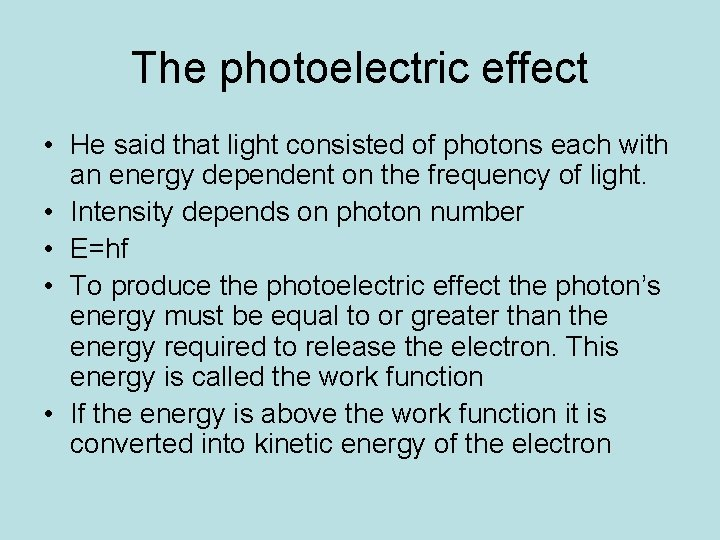The photoelectric effect • He said that light consisted of photons each with an energy dependent on the frequency of light. • Intensity depends on photon number • E=hf • To produce the photoelectric effect the photon’s energy must be equal to or greater than the energy required to release the electron. This energy is called the work function • If the energy is above the work function it is converted into kinetic energy of the electron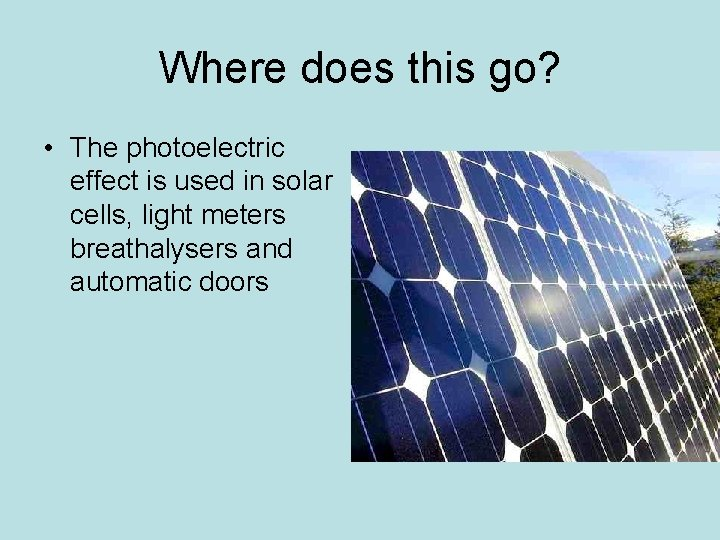Where does this go? • The photoelectric effect is used in solar cells, light meters breathalysers and automatic doors